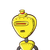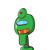# A rare whale fossil was uncovered under layers of sediment. When the excavator took measurements, she found the tail to be as long

A rare whale fossil was uncovered under layers of sediment. When the excavator took measurements, she found the tail to be as long as its head plus a quarter of the length of the body. When the archaeologist came in, he measured the length of the body. He found it to be ¾ of the total length. They made arguments about how such a fish would swim in the water. To make proper predictions they measured the head size, and found it to be 4 inches long. What is the total length of this whale?​

### 2 thoughts on “A rare whale fossil was uncovered under layers of sediment. When the excavator took measurements, she found the tail to be as long”

1.$$\Large\tt\fbox{Answer}$$

$$\sf{Let\:the\:total\:size\:of\:the\:whale\:be\:x}$$

$$\sf{Then,\:the\:size\:of\:the\:whale’s\:body\:is\: \frac{3}{4}x}$$

$$\sf{And\:the\:size\:of\:the\:whale’s\:tail\:is \: 4 + ( \frac{1}{4} \times \frac{3}{4} x)}$$

$$\bf\pink{According\:to\:the\:question:}$$

$$\sf{x – ( \frac{3}{4} + (4 + \frac{3}{16})) = 4}$$

$$\sf{⇒x – ( \frac{3}{4}x + 4 + \frac{3}{16}x) = 4}$$

$$\sf{⇒x – \frac{3}{4}x – 4 – \frac{3}{16} x = 4 }$$

$$\sf{⇒x – \frac{3}{4} x – \frac{3}{16} x = 4 + 4}$$

$$\sf{⇒ \frac{16x – 12x – 3x}{16} = 8}$$

$$\sf{⇒ \frac{x}{16} = 8}$$

$$\sf{⇒x = 8 \times 16}$$

$$\sf{⇒x = 128}$$

$$\bf\underline\red{Answer:}$$

The total size of the whale is 128 inches.

2.Step-by-step explanation:

$$\huge\sf\overbrace{Given:}$$

=> The tail is to be as long as it’s head plus a quarter of the length of the body.

=> Length of body be ¾ of the length.

=> Head is 4 inches long.

$$\huge\sf\overbrace{To \: Find :}$$

=>The total length of this whale

$$\huge\sf\overbrace{Solution:}$$

=> Body = x inch

=> Tail = Head + 1/4x

=> Tail = 4 + x/4 inches.

Total length = Head + Body + Tail

=> 4+x+4+x/4

=> 8 + 5x/4

Body = 3/4T Total length

x = 3/4 (8 + 5x/4)

4x = 3 × 32 + 5x/4

16x = 96 + 15x

x = 96

Total length = 8 + 5 × 96/4

=> 8 + 5 × 24

=> 8 + 120

=> 128

$$\huge\sf\overbrace{Answer:}$$